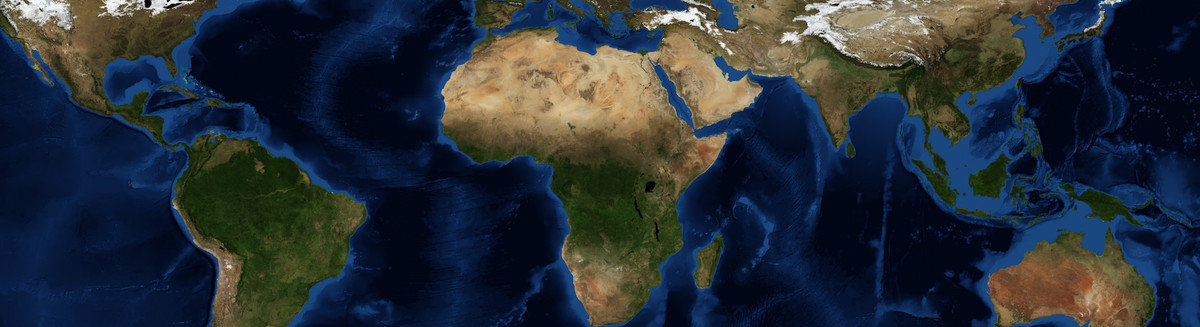This is a Preprint and has not been peer reviewed. The published version of this Preprint is available: https://doi.org/10.1080/13658816.2018.1511794. This is version 1 of this Preprint.

##### Abstract

There is a keen interest in inferring spatial associations between different variables spanning the same study area. We present a method for quantitative assessment of such associations in the case where spatial variables are either in the form of regionalizations or in the form of thematic maps. The proposed index of spatial association – called the V-measure – is adapted from a measure originally developed in computer science, where it was used to compare clusterings, to spatial science for comparing regionalizations. The V-measure is rooted in the information theory and, at its core, it is equivalent to mutual information between the two regionalizations. Here we re-introduce the V-measure in terms of spatial variance analysis instead of information theory. We identify three different contexts for application of the V-measure, comparative, associative, and derivative, and present an example of an application for each of them. In the derivative context, the V-measure is used to select an optimal number of regions for clustering-derived regionalizations. In effect, this also constitutes a novel way to determine the number of clusters for non-spatial clustering tasks as well. The advantage of V-measure over the Mapcurves method is discussed. We also use the insight from deriving the V-measure in terms of spatial variance analysis to point out a shortcoming of the Geographical Detector – a method to quantify associations between numerical and categorical spatial variables. The open-source software for calculating the V-measure accompanies this paper.

##### DOI

https://doi.org/10.31223/osf.io/rcjh7

##### Subjects

Categorical Data Analysis, Computer Sciences, Environmental Sciences, Geographic Information Sciences, Geography, Numerical Analysis and Scientific Computing, Physical Sciences and Mathematics, Social and Behavioral Sciences, Statistics and Probability, Theory and Algorithms

##### Keywords

clustering, Geographic Detector, mutual information, regionalization, spatial association

##### Dates

Published: 2018-04-20 04:16